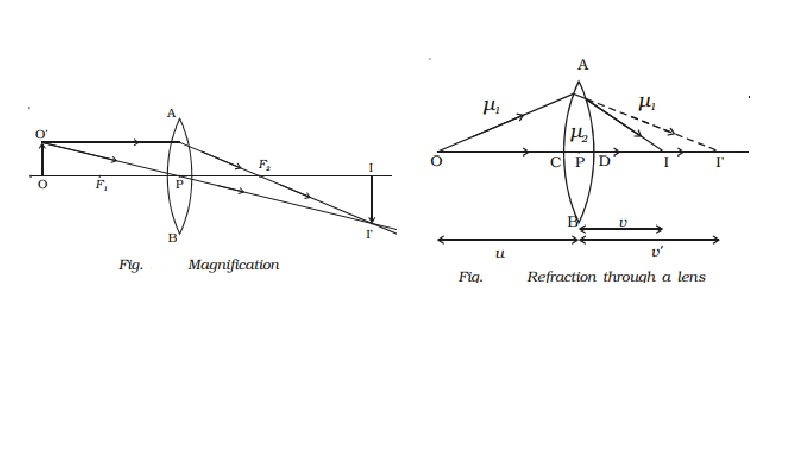Home | | Physics | | Physics | Lens maker's formula and lens formula and Magnification

# Lens maker's formula and lens formula and MagnificationLet us consider a thin lens made up of a medium of refractive index ?2 placed in a medium of refractive index ?1. Let R1 and R2 be the radii of curvature of two spherical surfaces ACB and ADB respectively and P be the optic centre.

Lens maker s formula and lens formula

Let us consider a thin lens made up of a medium of refractive index ?2 placed in a medium of refractive index ?1. Let R1 and R2 be the radii of curvature of two spherical surfaces ACB and ADB respectively and P be the optic centre.Consider a point object O on the principal axis. The ray OP falls normally on the spherical surface and goes through the lens undeviated. The ray OA falls at A very close to P. After refraction at the surface ACB the image is formed at I′. Before it does so, it is again refracted by the surface ADB. Therefore the final image is formed at I as shown in Fig.

The general equation for the refraction at a spherical surface is given by

( ?2/v ) ? (?1/u) = (?2-?1)/R       ????.(1)

For the refracting surface ACB, from equation (1) we write

?2/v?  - ?1/u  = (?2-?1)/R1  ????..(2)

The image I′ acts as a virtual object for the surface ADB and the final image is formed at I. The second refraction takes place when light

travels from the medium of refractive index ?2  to ?1.

For the refracting surface ADB, from equation (1) and applying sign conventions, we have

?1/v  - ?2/v? =[ (?2 - ?1)(-R2)]   ????(3)

. Adding equations (2) and (3)

?1/v  - ?2/u = (?2 - ?1 )[1/R1  - 1/R2]

Dividing the above equation by ?1

1/v -1/u = [(?2/ ?1)-1][ 1/R1 ? 1/R2 ]            ???????..(4)

If the object is at infinity, the image is formed at the focus of the lens.

Thus, for u = ∞, v = f. Then the equation (4) becomes.

1/f =  [(?2/ ?1)-1][ 1/R1 ? 1/R2 ]            ???????..(5)

If the refractive index of the lens is ? and it is placed in air, ?2  = ? and ?1  = 1. So the equation (5) becomes

1/f =  [?-1][ 1/R1 ? 1/R2 ]            ???????..(6)

This is called the lens maker?s formula, because it tells what curvature will be needed to make a lens of desired focal length. This formula is true for concave lens also.

Comparing equation (4) and (5)

We get 1/v ? 1/u = 1/f              ??..(7)

which is known as the lens formula.

Magnification

Let us consider an object OO ′ placed on the principal axis with its height perpendicular to the principal axis as shown in Fig.  The ray OP passing through the optic centre will go undeviated. The ray O ′A parallel to the principal axis must pass through the focus F2

. The image is formed where O ′PI′ and AF2 I′ intersect. Draw a perpendicular from I′ to the principal axis. This perpendicular II ′ is

the image of OO ′.The linear or transverse magnification is defined as the ratio of the size of the image to that of the object.

Magnification m = Size of the image / Size of the object = II?/OO? = h2/h1

where h1  is the height of the object and h2  is the height of the image.

From the similar right angled triangles OO′ P and II′ P, we have II?/OO? = PI/PO

Applying sign convention,

II′ = -h2

OO? = +h1

PI = +v

PO = -u

Substituting this in the above equation, we get magnification

M=-h2/+h1 = +v/-u

M=+ v/u

The magnification is negative for real image and positive for virtual image. In the case of a concave lens, it is always positive.

Using lens formula the equation for magnification can also be obtained as

m = h2/h1 = v//u = (f-v)/f = f/(f+u)

This equation is valid for both convex and concave lenses and for real and virtual images.

Study Material, Lecturing Notes, Assignment, Reference, Wiki description explanation, brief detail

Related Topics